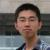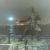关于多个线程使用同一个实例，是否影响执行速度

liunian324 发布于 2013/04/21 20:27

年薪达不到25W大数据工程师、拿不到Offer全额退款->>>OSChina 源码之 ActionServlet 控制类

package com.test;

import java.util.Random;

/**
*
*
*/
public class ThreadTest {
public static void main(String[] args) {
for (int i = 0; i < 10; i++) {
}
}
}

public String getComplexName(String name) {
long beginTime = System.currentTimeMillis();
System.out.println("***getComplexName(String name) name=" + name + " beginTime=" + beginTime);
int randomInt = 0;
for (int i = 0; i < 1000; i++) {// 为了该方法的执行时间足够长
for (int j = 0; j < 1000; j++) {
Random random = new Random();
randomInt = (randomInt + i + j + random.nextInt(100)) % 100;
}
}
long endTime = System.currentTimeMillis();
System.out.println("***getComplexName(String name) name=" + name + " beginTime=" + beginTime + " endTime=" + endTime + " costTime=" + (endTime - beginTime) + " randomInt=" + randomInt);

return name + "-Complex" + randomInt;
}
}

class ThreadDemo implements Runnable {

}

public void run() {
long beginTime = System.currentTimeMillis();
System.out.println("ThreadDemo " + threadName + " begin beginTime=" + beginTime);
long endTime = System.currentTimeMillis();
System.out.println("ThreadDemo " + threadName + " end beginTime=" + beginTime + " endTime=" + endTime + " costTime=" + (endTime - beginTime) + " complexName=" + complexName);
}
}

0ll

00
l

line:13 以及 line:15行

0
l

引用来自“yourOnlyJack”的答案

"楼主是问该代码有没有共享资源被多线程脏读的意思吧？？"

0
l

013行比15行好。结果一样的前提下，13比15行少创建9次对象，少分配9块内存。您说哪个好呀
0
l